Modelling of Non-Linear Pilot Disinfection Water System: A Bond Graph Approach

Naoufel ZITOUNI 1*, Ridha ANDOULSI 2*, Anis SELAMI 2, Abdelkader MAMI 3, and Hssen ABDENNASSEUR 4

1 Laboratory of Photovoltaic and the Semiconductors in CRTEn, BP95 Hammam lif 2050, Tunisia

2 Research Unit: Networks and Machines Electric in INSAT, Tunisia

3 Laboratory of automatic and control systems, Tunisia

4 Laboratory of Water and the Environment in CRTE, Tunisia

E-mails (*): naoufel_zitouni@yahoo.fr, Ridha.andoulsi@inrst.rnrt.tn; *Corresponding authors

Abstract

The ultraviolet (UV) irradiations are used to solve the bacteriological problem of the drinking water quality. A discharge-gas lamp is used to produce this type of irradiation. The UV lamp is fed by photovoltaic (PV) energy via electronic ballast composed by an inverter, a transformer and resonant circuit (RLC). The aim of this work is to give a useful global model of the system. In particular, we introduce the complicated UV lamp model and the water disinfection kinetics, where the radiant energy flux emitted by the discharge-gas lamp and the arc voltage are a complex functions of the current and time. This system is intended to be mainly used in rural zones, the photovoltaic modules as source of energy is an adequate solution.  To optimise the power transfer from the PV array to ballast and UV lamp, a Maximum Power Point Tracking (MPPT) device may be located between PV array and the loads. In this paper, we developed a bond-graph model which gives the water quality from UV flow, gas type, pressure, lamp current and geometrical characteristic. Finally reliable simulations are established and compared with experimental tests.

Keywords

Photovoltaic; MPPT; Ballast; A discharge-gas lamp; Disinfection water.

Introduction

The reuse of wastewater after adequate treatment constitutes a potential water resource which could be of great interest to several sectors, especially in countries suffering from a deficiency in water resource. Ultraviolet light has been shown to be very effective for the disinfection of drinking water and wastewater. The vast majority of existing UV disinfection systems employs low-pressure mercury arc lamps as their source of radiation. These lamps, which emit roughly 85% of their output power at a wavelength of 253.7 nm, have been shown to be highly effective for inducing photo biochemical changes in nucleic acids and proteins, without inducing significant photochemical changes in other constituents in treated water . The UV lamp is fed by electronic ballast composed by an inverter, a transformer and resonant circuit (RLC). The irradiation of water by a lethal dose allowed the accumulation of DNA photoproducts and the blockage of vital mechanisms of prosperity and viability of micro organisms such as DNA replication and RNA transcription, which ultimately result in reproductive of micro organisms’ inactivation.

Known that the determination of UV dose (the UV light dose is defined as UV intensity multiplied by time: duration of exposition water to UV irradiations) is one of interesting parameters allowing the accomplishment of optimal water disinfection [1-3]. A better control of a water quality can be achieved by developing a robust mathematical model. However modelling the disinfection kinetics is a difficult task due to the complexity of the treatment process. The complex physical, biological and chemical processes involved in wastewater treatment process exhibit non linear behaviours which are difficult to describe.  The UV lamp presents also a complicated model, indeed the radiant energy flux emitted by the discharge-gas lamp and the arc voltage are a complex functions of the current and time [4,5]. In fact, they depend on the lamp geometrical characteristics, gas type and pressure. We carried out a thorough study in order to found useful ballast, UV lump model and the water disinfection kinetics. Where  develops G-model of a discharge lamp,  use resistive approximation with three deviation from the linear model. In  the cubic approximation model and  develop a model with tangential approximation.

In this work we develop a mathematical model of the water treatment phenomenon which takes account of UV lamp, ballast, UV reactor and the current consumed. This model deduces water quality (N/N0) starting from UV flow and the various electric and mechanics quantities (dimension of engine UV).

Material and Method

The Schematic model and photo of the UV water disinfection system is shown in the figure 1.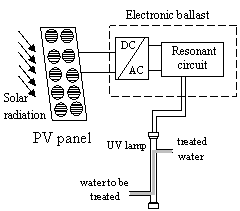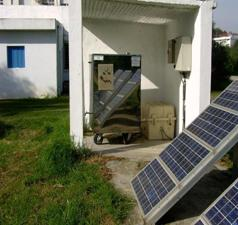Figure 1. Schematic model and photo of  the UV water disinfection system

The UV water disinfection system fed by a photovoltaic generator, object of our study, it consists of two tanks. A DC motor- pump ensures the circulation of water between the two tanks through UV engine. This latter, consists of a quartz sheath and a gas-discharge lamp low pressure. This type of lamp produce an irradiation wavelength of 265 nm induce a chemical reaction on the ARN and the AND of the micro-organism involving their deactivation.

Bond Graph Modelling of the UV Water Disinfection System

Modelling of water disinfection kinetics - The UV lamp model

All electric discharge lamps convert electrical energy into the light by transforming electrical energy into the kinetic energy of moving electrons, which in turn is converted into electromagnetic radiation as a result of some kind of collision process with atoms of gas (vapours) or their mixture.

Some moving electrons make such energetic impacts on atoms that the atoms internal state changes (inelastic collisions). These changes can be of two types: excitations or ionization.

Many models are based on the current-voltage characteristics of the discharge at high frequencies (> 20 Khz). They try to find some function that describe relation between voltage, current and power.

¸        Model with tangential approximation :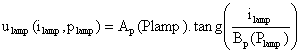(1)

where Ap(Plamp) and Bp(Plamp) are power functions that represent the steady state lamp behaviour. They are defined as polynomial functions where coefficients are determined from experimental data.

¸        Model use cubic approximation :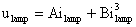(2)

where A, B are constants.

¸        Model use resistive approximation with three deviation from the linear model  (the dependence of the voltage-current characteristic on the lamp’s power level, the non linearity of the voltage-current curve at given operating condition and the time domain response of the lamp to a change in excitation). For a small power range is proposed equivalent resistance: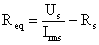(3)

where Us and Rs are lamp’s constant obtained from experimental data and Im is the current through the lamp.

¸        G- model of a discharge lamp [2, 3, 8]:

They describe a discharge conductance by a differential equation of the first order: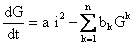(4)

where coefficients a, bk can be determined experimentally from the voltage-current characteristics or from physical evaluation is possible when n is 2 or 3. In this case have coefficients physical meaning.

An electric conductivity of the discharge can be calculated from: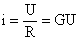(5)

where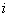is current through discharge, U is discharge voltage, R is resistivity and G is the electric conductivity of the discharge.

The polynomial model can be used with n=2 with good results. The constants a, b1, and b2 would have physical meaning in this case. The model developed by [3,7] has the following form: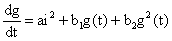(6)

The lamp voltage and the lamp conductance are obtained when the differential equation corresponding to the model is solved. The input current provided by the electronic ballast i(t), is known.

There are several alternatives of UV lamp modelling, we retain the G-model. It can be seen that the polynomial model also fits slightly better than the exponential model at high frequency .

Starting of the Lamp

A gas-discharge lamp needs a sufficient voltage for starting. This lamp uses ballast able to create a high frequency voltage and an increase in the voltage value for a short time on the UV lamp. This over voltage is obtained by a resonant circuit (RLC). The voltage delivered by converter DC/AC, feed a transformer towards a resonant system formed by a resistance Rr in series with an  inductance Lr and two capacity Cr1  and Cr2 (Figure 2 ). The receiver is a gas-discharge lamp (UV) which is compared to infinite impedance before starting and low value impedance after starting.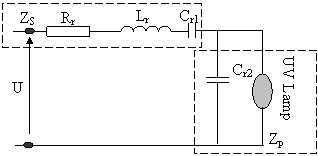Figure 2. Electric schema of UV lamp coupled to the ballast

Modelling of Water Disinfection Kinetics

The absorption of UV radiations is directly related to the molecule structure and particularly to the organic groupings operating. The law describing this phenomenon is given by Beer Lambert expression :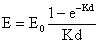(7)

where: E = lamp intensity (μw/cm2); E0 = Initial intensity (μw/cm2); d = Thickness of the fluid or optical way (cm); K = Absorption coefficient (cm-1) which is often given or identified.

The light bactericidal effectiveness is related to the exposure amount. The UV dose radiation (D) in a point r of engine, is the product of the radiation intensity (illumination) in this point (Er) and the contact time in the engine (tc):

Dr = Er tc                                                                                                        (8)

with: Dr =UV dose at the point r (mW·s·cm-2); Er = Intensity UV at the point r (mW·s·cm-2); tc = contact time in second.

The Specific energy necessary to the total or partial destruction of the micro-organisms is function of the physical-chemical composition of treated water and the germs sensitivity. A modelling study has been carried out under conditions well controlled on a laboratory photo engine and stocks of Pseudomonas aeruginosa. This study shows that the inactivation kinetics of these bacteria corresponds well to the modified model of Chick Watson :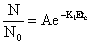(9)

with: N = number of micro-organisms; N0 = initial number of micro-organisms; K1 = coefficient translating the potent capacity disinfecting which depends on the type on micro-organisms; A = initial abatement of the germ number.

The parameters A and K1 are given from experiments.

The radiant energy flux, emitted by a discharge-gas lamp, depends on the geometrical characteristics of the lamp, the gas type, the pressure, temperature. This dependence makes difficult the development of the general model characterizing the variation of emitted flux or the arc voltage according to the arc current and time.  The relations obtained in this field are given in an empirical method.

In literature, it is shown that between 50 % and 60 % of the power consumed by an UV discharge-gas lamp low pressure is transformed into UV ray [1, 2]:

F0 = βP                                                                                                          (10)

where F0 = the luminous flow emitted by the lamp; P = power consumed by the lamp.

Influence of the Flow Rate and Illumination on the Water Disinfection

The illumination measured very close to the lamp surface corresponds to the greatest value in the reactor. In a mono lamp reactor, the lamp protected by a quartz tube is placed in the axis of engine. The general diagram of water disinfection engine is given by the Figure 2.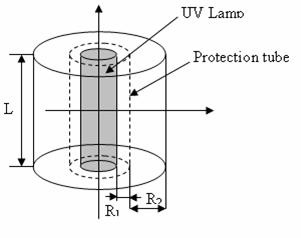Figure 2. Schema of the UV reactor

In this study we consider that all the fluids molecules cross the following reactor with parallel trajectories and at the same speed.  In this laminar flow there is neither mixture nor dispersion, all the particles have even time of residence equal to the average residence hydraulic time. The average amount deposited in the reactor is defined as being the relationship between the total energy deposited in the engine volume per second and the volume of fluid which crosses the entire reactor per second. The illumination varies according to the equation (11):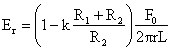(11)

where k = constant which is worth 0.1 for our case; L = Length of UV lamp.

The speed in any point of the engine vr is equal to the speed in the centre of the reactor v0 (vr = v0), under these conditions the fraction of the flow subjected to illumination is modelled by equation (12):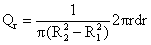(12)

Therefore the average value of the balanced illumination of the luminous flows is written in the following form: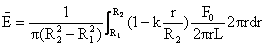(13)

According to the equation above, we can connect the ratio of water disinfection according to the flow rate Q, luminous flow F0 and consequently the operating ballast frequency. This relation permits also to improve the results of water disinfection either on the flow rate or on luminous flow according to a data base.  This relation can be summarized in the equation system (14):(14)

Results and discussion

Bond Graph Modelling of the UV Water Disinfection Reactor

The ultraviolet irradiation presents a particular solution for the disinfection of water. A gas-discharge lamp necessities a sufficient tension for starting. This lamp uses ballast able to create a high frequency tension and an increase in the voltage value for a short time on the UV lamp. The power supply of the lamp (ballast) is an inverter followed by a transformer that transforms the tension 24V provided by the battery into high frequency sinusoidal tension. This one feeds a serial RLC resonant circuit to increase the voltage of the UV lamp. Before the starting up of the discharge-gas lamp, the lamp has infinite impedance. After the starting up, this impedance becomes low [10,11]. The BG model of the UV system is given by Figure 3.

The BG model of the inverter required a raise in the simulation time; to simplify the model and to decrease the time of simulation we use the average BG method. When the BG model of the inverter is established, the remainder of the circuit is easily deduced.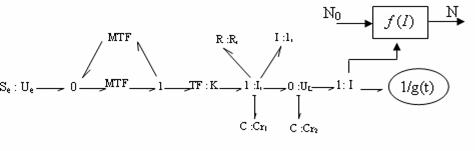Figure 3. Bond graph model of the UV system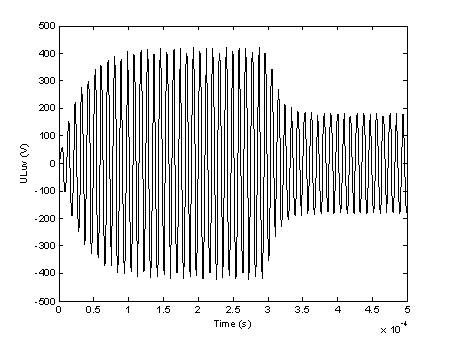Figure 4. Simulation of the bond-graph model of the ballast output voltage

(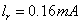,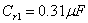,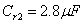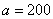,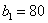,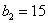)

Simulation shows (Fig. 4) that the ballast transforms the voltage 24V provided by the battery into high frequency tension and an increase in the voltage value for a short time on the UV lamp. Once the lamp is started, the voltage on its terminals decreases allowing a nominal operation of UV reactor.

Bond-Graph Modelling of the Photovoltaic Source

The UV lamp is fed by photovoltaic source. The PV generator has a non-linear current-voltage (I-V) characteristic. To simulate (I-V) characteristic, we use the bond-graph model corresponding to the simplified equivalent circuit of PV generator represented in figure 5 [12-14].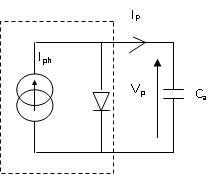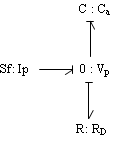a b

Figure 5. Equivalent scheme of simplified PV array model (a) and its Bond graph model (b)

In order to test the theoretical model, we had compared the experimental (I-V) characteristic of the PV array to the corresponded of BG simulated one. The two curves are shown in Figure 6.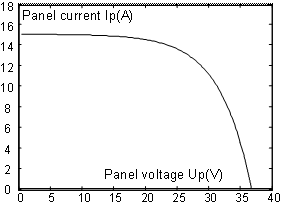Figure 6. Simulated (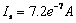,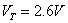,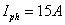) (a) and experimental (b)  (I-V) characteristic of the PV array.

The Bond-Graph Modelling of DC/DC Converter

The DC/DC Converter Model

When the PV generator is directly connected to the load, the system will operate at the intersection of the (I-V) curve and load line, which can be far from the maximum power point MPP.A proper matching between the PV generator and the electric load should be considered. Therefore the coupling between the ballast + UV lamp and the PV module is implemented via a Maximum Power Point Tracking controller to operate the PV system at its maximum output power for any temperature and solar radiation level. The tracking algorithm control of the MPPT is developed in another work .

The converter consists of power components and control circuit. A control algorithm permits to assure maximum output power. We will use the buck converter for the MPPT controllers used in this case. These controllers are used to minimize the error between the operating power and their reference (corresponding to the maximum power).The equivalent scheme of DC/DC converter is presented in Fig. 7.Figure 7. Scheme of the buck converter

The Bond-Graph Modelling of DC/DC Converter

This method supposes the ideal switches and gives indirectly the average models appropriate to the various static inverters which lead to deduce easily the bond-graph model. For the case of the buck converter, the average values of electric parameter are given by equation (14) and (15) [14, 15].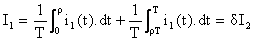(14)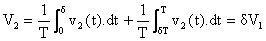(15)

There are five steps used to construct the averaged bond graph model, it is detailed in . The averaged bond graph model of a buck converter developed in  is illustrated in the Figure 8.Figure 8. The bond-graph model of buck converter

The complete BG model of the system is obtained by associating the models developed previously. The global model is represented by the Figure 9.

After elaborating the global BG model of treatment water system, a thorough study can be done. Indeed, from this model, we can simulate the lamp current, voltage and power as well as the response in bacteriological attack according to UV dose radiation. These simulations are shown, respectively, in Figure 10 and Figure 13. The experimental results are represented by Figure 11, 12 and 13.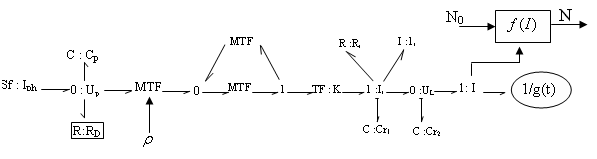Figure 9. Global bond-graph model of water disinfection system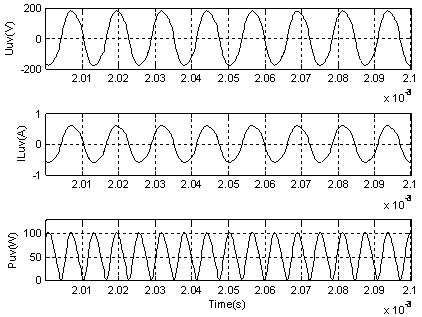Figure 10. Simulation of the lamp current, voltage and power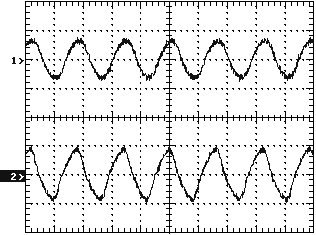Figure 11. I-lamp (curve 1; 1A/Div -10ms/Div), U-lamp (curve 2; 200V/Div-10ms/Div)) f = 64KHz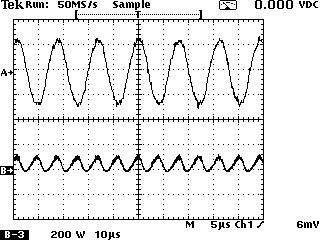Figure 12. I-lamp (curve 1; 0.5A/Div -10ms/Div), P-lamp (curve 2; 200W/Div-10ms/Div

According to simulations the lamp current and voltage have a high frequency sinusoidal form. The power presents a double alternation form since it is equal to the product of the lamp voltage and the current. It is noticed that the power frequency is doubled. In order to test the theoretical model, we had compared the simulation, corresponding to BG model established, to the experimental result the curves are shown in the following figure. The starting voltage and waveforms of the UV lamp are shown in figure.4, where we observe that the peak voltage is approximately 400V. The UV lamp steady-state voltage and current waveforms, with the inverter operating at 100 Khz are shown in figure.8, where the UV lamp voltage effective value is approximately equal to 180V, current effective value approximately 0.8A and output power approximately 80W. In order to confirm the established bond graph model and the obtained simulations, we belonged to experimental measurements of the UV lamp voltage, current and power. The curves obtained are identical to simulations.

We can say that the experimental results confirm the elaborated simulations for the lamp current, voltage, pressure and bacteriologic attack response. The complete model obtained represented the operation of the pilot unit water treatment starting from generator statement until the quality of water translated by expression N/N0. One being based with bacteriological analyses, we can act on the quality of water from the model obtained in spite of the complexity of the phenomenon of water treatment.

Conclusion

This study proposes a treated water system by UV irradiation using discharge-gas lamp, ballast and photovoltaic generator. In this paper, at first step, the model of UV discharge-gas lamp (height frequency) is developed. In the second step, we developed the water disinfection kinetics. We proposed a unified approach of modelling based on graphic technique known as bond-graph. This technique is systematic and has a sufficient flexibility to be able to introduce various components into the system. In the developed model we connected the parameters which act on the water treatment phenomenon (water disinfection) by UV irradiation, such as the UV lamp illumination, the consumed lamp current, dimensions of the quartz sheath.

For the developed BG models, we represented the simulation results carried out in the 20.Sim environments. In simulations, we showed different phenomenon which governs the system operation such as the nonlinear (I-V) characteristic, the operation of the ballast and UV reactor, the response in bacteriological attack according to UV dose radiation (the water disinfection kinetics. To validate the models carried out we have to compare simulations obtained with the experimental results. A control strategy of the system can be implemented in order to improve the quality of water.

References

1.      Abdennaceur H., Mabrouk M., Ouazri H., Cherif M., Boudabous A., Damelincourt J.J., UV disinfection of treated waste water in a large-scale pilot plant and inactivation of selected bacteria in a laboratory UV device, Bioresource Technology 2000, 74(2), 141-150.

2.      Zissis G., Damelincourt J.J., Modelling discharge lamps for electronic circuit designers: A review of the existing methods. Plasma Science, 2002. ICOPS 2002. IEEE Conference Record - Abstracts. The 29th IEEE International Conference on 2002; DOI: 10.1109/PLASMA.2002.1030646.

3.      Rossat-Mignod P., Rojat G., Joubert Ch., Zassis J., Constraints generated by fluorescents lamps on the electrical network, Industry Applications Conference, 2002. 37th IAS Annual Meeting, 2002, 1, p. 355-362.

4.      Ben-Yaakov S., Shavatsas M., Glozman S., Statics and dynamics of fluorescent lamps operating at high frequency: modeling and simulation, Applied Power Electronics Conference and Exposition, 1999. APEC '99. Fourteenth Annual, 1999, 1, p. 467-472.

5.      Ribarich T.J., Ribarich J.J., A new high-frequency fluorescent lamp model, Industry Applications Conference, 1998. Thirty-Third IAS Annual Meeting. The 1998 IEEE 1998, 3, p. 2094-2098.

6.      Gervi M., Fortes E.C., Seidel A.R., Bisogno F.E., do Prado R.N., Fluorescent lamp model employing tangent approximation, Power Electronics Specialists Conference, PESC 2002, IEEE 33rd Annual, 2001, 1, p. 187-191.

7.      Zheng S., Conductance Calculation - Molecular Flow, Long Tube of Circular Cross Section. GEM TN-93-382 (USDE document, 5p.). 1993.

8.      Zitouni N., Andoulsi R., Sellami A., Mami A., Hssen A., Sliding mode control for non linear water treatment system, Systems Signals and Devices (SSD), 2010 7th International Multi-Conference on 2010; doi: 10.1109/SSD.2010.5585546.

9.      Viejo C.B., Anton J.C.A., Robles A., Martin F.F., Viera J.C., Bhosle S., Zissis G., Comparison between Different Discharge Lamp Models Based on Lamp Dynamic Conductance, Industry Applications, IEEE Transactions, 2011, 47(4), p. 1983-1991.

10.  Jui P.Y., Horng C.H., Design and testing of a separate type lighting system using solar energy and cold-cathode fluorescent lamps, Applied Energy, 2007, 84(1), p. 99-115.

11.  Felix G.R., Lifetime evaluation of DC-supplied electronic ballast with fluorescent lamps for photovoltaic applications, Renewable Energy, 2004, 29(6), p. 961-974.

12.  Borowy B.S., Salameh Z.M., Dynamic response of a stand-alone wind energy conversion system with battery storage to a wind gust, Energy Conversion, IEEE Transactions 1997, 12(1), p. 73-78.

13.  Sukamongkol Y., Chungpaibulpatona S., Ongsakul W., A simulation model for predicting the performance of a solar photovoltaic system with alternating current loads, Renewable Energy, 2002, 27(2), p. 237-258.

14.  Andoulsi R., Mami A., Dauphin-Tanguy G., Annabi M., Bond graph modelling and dynamic study of a photovoltaic system using MPPT buck-boost converter. Systems, Man and Cybernetics, 2002 IEEE International Conference on 2002, 3, doi: 10.1109/ICSMC.2002.1176036.

15.  Zitouni N., Andoulsi R., Sellemi A., Mami A., Hssen A., A new Bond Graph Model of a Water Disinfection System Based on UV Lamp Feed by Photovoltaic Source: Simulation and Experimental Results, Journal of Automation & Systems Engineering, 2011, 5(2), p. 79-95.

16.  Mezghanni D., Andoulsi R., Mami A., Dauphin-Tanguy G., Bond graph modelling of a photovoltaic system feeding an induction motor-pump, Simulation Modelling Practice and Theory, 2007, 15, p. 1224-1238.